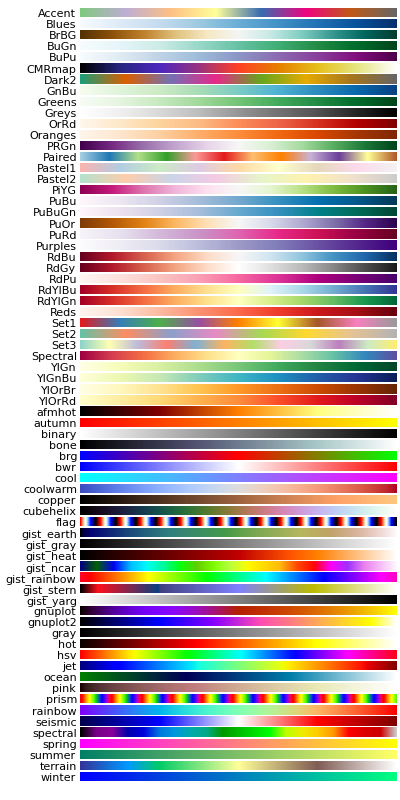You are reading an old version of the documentation (v1.2.1). For the latest version see https://matplotlib.org/stable/# pylab_examples example code: show_colormaps.py¶```# This example comes from the Cookbook on www.scipy.org.  According to the
# history, Andrew Straw did the conversion from an old page, but it is
# unclear who the original author is.
import numpy as np
import matplotlib.pyplot as plt

a = np.linspace(0, 1, 256).reshape(1,-1)
a = np.vstack((a,a))

# Get a list of the colormaps in matplotlib.  Ignore the ones that end with
# '_r' because these are simply reversed versions of ones that don't end
# with '_r'
maps = sorted(m for m in plt.cm.datad if not m.endswith("_r"))
nmaps = len(maps) + 1

fig = plt.figure(figsize=(5,10))
fig.subplots_adjust(top=0.99, bottom=0.01, left=0.2, right=0.99)
for i,m in enumerate(maps):
ax = plt.subplot(nmaps, 1, i+1)
plt.axis("off")
plt.imshow(a, aspect='auto', cmap=plt.get_cmap(m), origin='lower')
pos = list(ax.get_position().bounds)
fig.text(pos - 0.01, pos, m, fontsize=10, horizontalalignment='right')

plt.show()
```

Keywords: python, matplotlib, pylab, example, codex (see Search examples)